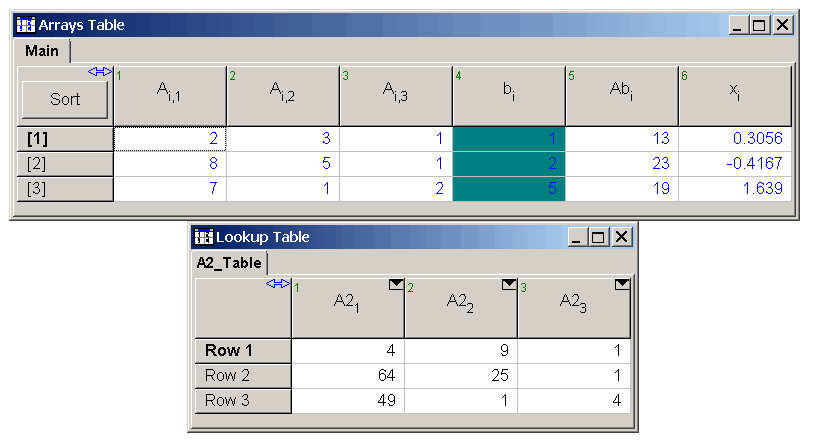Contents - Index

MATLAB. (Macro Commands)

MATLAB.Open

Start MATLAB and open the MATLAB Command Window.   This command is ignored if MATLAB has been previously opened in this sessionn and not closed with the MATLAB.Quit command.

MATLAB.Execute(MATLAB Command)

Execute the MATLAB command. Note the MATLAB variables and commands are case-sensitive. The command can

be a string constant or a string variable.  The command is executed in the MATLAB command window.  The MATLAB command is case-sensitive.  It must be entered exactly as it would be entered in the MATLAB Command Window.  The result of the command is displayed in the macro log file.  Note that MATLAB.Open command must be used before this command can be issued.

Example:  MATLAB.Execute(x=A\b).  {Assumes matrix A and vector b are defined in MATLAB}

EESVarName=MATLAB.GetVariable(MLVarName)

Retrieve an existing MATLAB variable defined in the MATLAB 'base' workspace.  The MATLAB variable may be a single real value, a single string variable, a 1-D MATLAB vector or a 2-D MATLAB array.  Note that MATLAB.Open command must be used before this command can be issued.  If a vector or array is used, the dimensions provided in EESVarName must be the same size as those for the MATLAB variable.

Example 1:

MATLAB.Execute(HW='Hello World!')

HW\$=MATLAB.GetVariable(HW)

Example 2:

MATLAB.Execute(x=sqrt(36))

x=MATLAB.GetVariable(x)

Example 3:

{assumes vector b is defined in MATLAB}

b[1..3]=MATLAB.GetVariable(b).

Example 4:

MATLAB.Execute(A=[1 2 3; 4 5 6; 7 8 9])

N=3

A[1..N,1..N]=MATLAB.GetVariable(A)

LookupTableName=MATLAB.GetTable(MLVarName)

Retrieve an existing MATLAB vector or array defined in the MATLAB 'base' workspace and copy the values into an EES Lookup table.  The LookTableName may be provided as a string variable, e.g., LookupTableName\$.  The MATLAB variable may be a 1-D MATLAB vector or a 2-D MATLAB array.  Note that MATLAB.Open command must be used before this command can be issued.  If a vector or array is used, the dimensions provided in EESVarName must be the same size as those for the MATLAB variable.

Example 1:

MATLAB.Execute(b=[1 2 3])

LookupRow=MATLAB.GetTable(b)

Example 2:

MATLAB.Execute(b=[1; 2; 3])

LookupCol=MATLAB.GetTable(b)

Example 3:

MATLAB.Execute(A=[1 2 3; 4 5 6; 7 8 9])

myLookupTable\$='A'

myLookupTable\$=MATLAB.GetTable(A)

MATLAB.SetVariable(MLVarName,EESVarName)

Sets the value of MATLAB variable, MLVarName, to the value an existing and defined EES variable, EESVarName. MLVarName may be a  single real value, a single string variable, a 1-D MATLAB vector or a 2-D MATLAB array. The EESVarName must be of the same type (real or string).  If EESVarName is a vector or array, the dimensions must be explictly defined.

Example 1:

S\$='Hello!  Welcome to EES and MATLAB'

MATLAB.SetVariable(aStr,S\$)

Example 2:

brow[1,1..3]=[1,2,3]  {Set a row vector}

MATLAB.SetVariable(brow,brow[1,1..3])

bcol[1..3,1]=[1,2,3]  {Set a column vector}

MATLAB.SetVariable(bcol,bcol[1..3,1])

b[1..3]=[1,2,3]

MATLAB.SetVariable(bcol,b[1..3])  {This also sets a column vector}

Example 3:

N=3

a[1,1..N] = [2, 3, 1]

a[2,1..N] = [8, 5, 1]

a[3,1..N] = [7, 1, 2]

MATLAB.SetVariable(A,A[1..N,1..N])

MATLAB.Quit

Close the instance of MATLAB opened with the MATLAB.Open macro command.  Note that MATLAB must be reopened if it is used after this command has executed.  The MATLAB.Open process is slow, so only close MATLAB when you are done using it.

Note the the MATLAB macro commands can be used within the \$RunMacroAfter directive, as shown in the foillowing example.

N=3

A[1,1..N] = [2, 3, 1]

A[2,1..N] = [8, 5, 1]

A[3,1..N] = [7, 1, 2]

b[1..N] = [1, 2, 5]

\$RunMacroAfter

MATLAB.Open

MATLAB.SetVariable(A,A[1..N,1..N])

MATLAB.SetVariable(b,b[1..N])

MATLAB.Execute(X=A\b)

x[1..N]=MATLAB.GetVariable(X)

MATLAB.Execute(Ab=A*b)

Ab[1..3]=MATLAB.GetVariable(Ab)

MATLAB.Execute(A2=A.*A)

A2_Table=MATLAB.GetTable(A2)

MATLAB.Quit

ShowWindow Arrays

ShowWindow LookupTable

\$endmacroMacroCommands